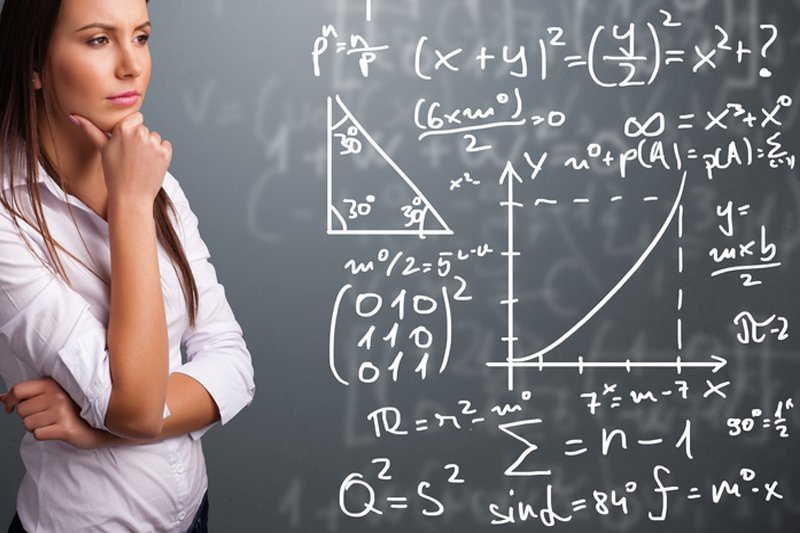## College Algebra

##### Overview

College Algebra is equivalent to a 1-semester, college level course that focuses on the general topics of Algebra.
This course is available live and on-demand through the online Nursing , Other Degree , and Individual Course Credits programs.

• All Other Degrees

Course Type: Credit-By-Course
Credits: 3 Semester Credits
Category: Natural Science and Mathematics
Course Equivalence: College-Level Mathematics or General Elective
Length: 10 Lectures + 1 Final Exam
Assessments: : Weekly Quizzes and Final Exam (90 mins | 60 Questions)

College Algebra is a college-level algebra course for non-mathematics majors. In this course, students will become familiar with currently taught algebraic vocabulary, symbols, and notation and will learn to solve both routine problems requiring basic algebraic skills and non-routine problems that demonstrate their understanding of concepts. The subject matter of this course includes number systems and operations, algebraic operations, equations and inequalities, and functions and their properties.

#### Course Objectives

• Understand basic algebraic operations such as exponents, factoring, absolute value, and properties of logarithms;
• Interpret functions in forms that include graphs, numerals, and symbols;
• Identify and analyze number systems and their operations including sequences, series, and factorials;
• Apply algebraic knowledge to both routine (straightforward) and non-routine (real world) problems.
• Solve linear and quadratic equations and inequalities

• Nursing

Course Type : Credit-By-Exam
Credits: 3 Semester Credits
Category: Natural Science and Mathematics
Course Equivalence: College-Level Mathematics or General Elective
Length: 10 Lectures + 1 Final Exam
Assessments : 1 Exam at a CLEP® Testing Facility (90 mins | 75 Questions)

This course prepares individuals for a credit-by-exam that focuses on currently taught algebraic vocabulary, symbols, and notation. Students will learn to solve both routine problems requiring basic algebraic skills and non-routine problems that demonstrate their understanding of concepts. The subject matter of the exam includes number systems and operations, algebraic operations, equations and inequalities, and functions and their properties.

#### Course Objectives

• Understand basic algebraic operations such as exponents, factoring, absolute value, and properties of logarithms;
• Interpret functions in forms that include graphs, numerals, and symbols;
• Identify and analyze number systems and their operations including sequences, series, and factorials;
• Apply algebraic knowledge to both routine (straightforward) and non-routine (real world) problems.
• Solve linear and quadratic equations and inequalities